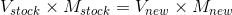In: Chemistry

# Calculate how to prepare 500 mL of 32.5 part per million KOH from a solution 50%...

Calculate how to prepare 500 mL of 32.5 part per million KOH from a solution 50% of KOH with a density of 1.58 g/mL

## Solutions

##### Expert Solution

1 ppm is, in terms of percentage is essentially 1g of the substance in 1000000g of the solution. So, 1 g/mL is equal to 1000000 ppm.

Now, given that the stock solution contains 50% KOH or 0.5 g/mL and that its density of the 50% KOH is 1.58 g/mL, it can be said to contain 500000 ppm of KOH. Also, 32.5 ppm, in terms of percentage will then be 32.5x10-6 g/mL.

Using the law of equivalence for dilution, we get,This on substitution gives 500*(32.5x10-6) = X*0.5

The unknown X amounts to 500*(32.5x10-6)/0.5 = 0.0325 mL.

So, taking 0.0325 mL of the 50% KOH solution and diluting it to 500 mL will produce a solution contain 32.5 ppm of KOH.

## Related Solutions

##### A 50.0 mL solution of 0.167 M KOH is titrated with 0.334 M HCl . Calculate...
A 50.0 mL solution of 0.167 M KOH is titrated with 0.334 M HCl . Calculate the pH of the solution after the addition of each of the given amounts of HCl . 0.00 mL pH = 7.00 mL pH = 12.5 mL pH = 19.0 mL pH = 24.0 mL pH = 25.0 mL pH = 26.0 mL pH = 31.0 mL pH =
##### *Calculate the mass of sodium acetate (CH3COONa) required to prepare 50 mL of a 0.10 M...
*Calculate the mass of sodium acetate (CH3COONa) required to prepare 50 mL of a 0.10 M sodium acetate solution. Show work. Verify that this matches the amount you place into cup #3 in Activity 1. *Calculate the volume of 6 M acetic acid needed to prepare 100 mL of a 0.10 M acetic acid (CH3COOH) solution. Show work. *Calculate the volume of 1 M sodium hydroxide (NaOH) needed to prepare 10 mL of a 0.10 M sodium hydroxide solution. Show...
##### How many 2 g tablets are needed to prepare 100 mL of a​ 10% solution?
How many 2 g tablets are needed to prepare 100 mL of a​ 10% solution?
##### how manu mL of 5.00 M NaOH solution are needed to prepare 750mL of a 0.150...
how manu mL of 5.00 M NaOH solution are needed to prepare 750mL of a 0.150 M NaOH solution? and how many mL of water misy be added to prepare the above solution?
##### Calculate the pH of a 0.0204 M KOH solution at each temperature. For 15 (4.57 ×...
Calculate the pH of a 0.0204 M KOH solution at each temperature. For 15 (4.57 × 10–15                                                                                                                                                     15 °C pH=                      For 45 (3.94 × 10–14) 45 °C pH=
##### A solution with a final volume of 500 mL was prepared by dissolving 25 mL of...
A solution with a final volume of 500 mL was prepared by dissolving 25 mL of methanol {CH2OH} (density = 0.7914) in chloroform {CHCl3}. Calculate its molar concentration. (MWMethanol = 32.00) (show the solution)
##### 25.0 mL of a 0.100 M HCN solution is titrated with 0.100 M KOH. Ka of...
25.0 mL of a 0.100 M HCN solution is titrated with 0.100 M KOH. Ka of HCN=6.2 x 10^-10. a)What is the pH when 25mL of KOH is added (this is the equivalent point). b) what is the pH when 35 mL of KOH is added.
##### The following buffer was prepared: 50. mL 2.0 M methylamine (CH3NH2) solution and 50. mL of...
The following buffer was prepared: 50. mL 2.0 M methylamine (CH3NH2) solution and 50. mL of 2.0 M methylamine hydrochloride (CH3NH3Cl) solution. The Kb for methylamine is 4.4 x 10-4. What is the pH of the resulting solution after adding 100 mL of 1.0 M KOH to the original buffer solution?
##### Calculate the pH of the solution that results from each of the following mixtures. 140.0 mL...
Calculate the pH of the solution that results from each of the following mixtures. 140.0 mL of 0.27 M  HF with 225.0 mL of 0.30 M  NaF 175.0 mL of 0.12 M C2H5NH2 with 265.0 mL of 0.20 M C2H5NH3Cl Express your answer using two decimal places.
##### 78 A.) Calculate the pH of the solution that results from mixing 20.0 mL of 0.022...
78 A.) Calculate the pH of the solution that results from mixing 20.0 mL of 0.022 M HCN(aq) with 80.0 mL of 0.066 M NaCN(aq). pH= b. Calculate the pH of the solution that results from mixing 28.0 mL of 0.117 M HCN(aq) with 28.0 mL of 0.117 M NaCN(aq). C. Calculate the pH of the solution that results from mixing 66.0 mL of 0.057 MHCN(aq) with 34.0 mL of 0.034 M NaCN(aq).. The Ka value for HCN is 4.9×10^(−10)...